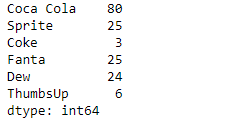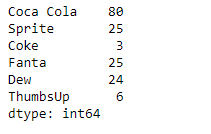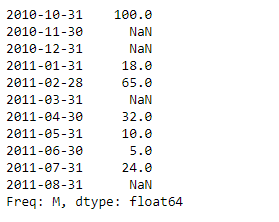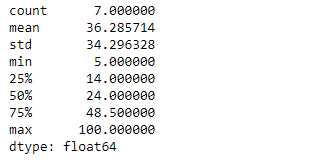# Python | Pandas Series.describe()

• Last Updated : 15 Feb, 2019

Pandas series is a One-dimensional ndarray with axis labels. The labels need not be unique but must be a hashable type. The object supports both integer- and label-based indexing and provides a host of methods for performing operations involving the index.

Pandas` Series.describe()` function generate a descriptive statistics that summarize the central tendency, dispersion and shape of a dataset’s distribution for the given series object. All the calculations are performed by excluding NaN values.

Syntax: Series.describe(percentiles=None, include=None, exclude=None)

Parameter :
percentiles : The percentiles to include in the output.
include : A white list of data types to include in the result. Ignored for Series.
exclude : A black list of data types to omit from the result. Ignored for Series

Returns : Summary statistics of the Series

Example #1: Use `Series.describe()` function to find the summary statistics of the given series object.

 `# importing pandas as pd ` `import` `pandas as pd ` ` `  `# Creating the Series ` `sr ``=` `pd.Series([``80``, ``25``, ``3``, ``25``, ``24``, ``6``]) ` ` `  `# Create the Index ` `index_ ``=` `[``'Coca Cola'``, ``'Sprite'``, ``'Coke'``, ``'Fanta'``, ``'Dew'``, ``'ThumbsUp'``] ` ` `  `# set the index ` `sr.index ``=` `index_ ` ` `  `# Print the series ` `print``(sr) `

Output :Now we will use `Series.describe()` function to find the summary statistics of the underlying data in the given series object.

 `# find summary statistics of the underlying  ` `# data in the given series object. ` `result ``=` `sr.describe() ` ` `  `# Print the result ` `print``(result) `

Output :As we can see in the output, the `Series.describe()` function has successfully returned the summary statistics of the given series object.

Example #2 : Use `Series.describe()` function to find the summary statistics of the underlying data in the given series object. The given series object contains some missing values.

 `# importing pandas as pd ` `import` `pandas as pd ` ` `  `# Creating the Series ` `sr ``=` `pd.Series([``100``, ``None``, ``None``, ``18``, ``65``, ``None``, ``32``, ``10``, ``5``, ``24``, ``None``]) ` ` `  `# Create the Index ` `index_ ``=` `pd.date_range(``'2010-10-09'``, periods ``=` `11``, freq ``=``'M'``) ` ` `  `# set the index ` `sr.index ``=` `index_ ` ` `  `# Print the series ` `print``(sr) `

Output :Now we will use `Series.describe()` function to find the summary statistics of the underlying data in the given series object.

 `# find summary statistics of the underlying  ` `# data in the given series object. ` `result ``=` `sr.describe() ` ` `  `# Print the result ` `print``(result) `

Output :As we can see in the output, the `Series.describe()` function has successfully returned the summary statistics of the given series object. `NaN` values has been ignored while calculating these statistical values.

My Personal Notes arrow_drop_up
Recommended Articles
Page :